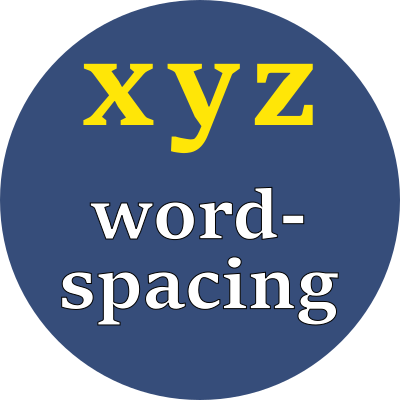# word-spacing## Property values

Use any of these font-relative typographic units:

 % percentage A percentage of the current font's advance measure (the width) em em The `font-size` of the current font rem relative em The `font-size` of the element ch "0" The advance measure (the width) of the current font's "0" glyph ic ideograph count The advance measure (the width) of the current font's "水" ideograph

Or use any of these fixed size units which are independent of the current font:

 in inch A size equal 96px px pixels A size equal to 1/96 inch pt point A size equal 1in/72 = 1.33px pc pica A size equal 1in/6 = 12pt = 16px cm centimeter A size equal to 96px/2.54 = 37.8px mm millimeter A size equal to 96px/25.4 = 3.8px Q quarter millimeter A size equal to 96px/1016 = 0.94px

## Examples

style > character > word-spacingWhitespace effect for words